# Types of Slide Rules and their Scales

All slide rules consist of logarithmic scales that can be moved in relation to each other in order to do basic mathematical calculations. The simplest slide rule would have two log scales that you could use to do multiplication and division (see my Slide Rule Introduction page for more info). One of the influential figures in slide rule design, Amédée Mannheim, created a standard slide rule with 4 scales labelled A, B, C, D, in that order (the C/D scales are the standard length log scales). These labels are hardly very descriptive, but they caught on and were perpetuated right up until the end of slide rule production.

As time went by, increasingly specialized scales were added to the rules, increasing the complexity and capabilities of the device. Unlike the basic standard scales, these typically were assigned labels that reflected their function, albeit in a limited way (e.g. K for cubic scales, since C was already taken). Toward the end of slide rule production, many makers began including additional "self-documenting" features at one end of the scales, illustrating the mathematical relationship of each scale to the basic C/D scales. Clearly, this was a great relief for beginners, as deciphering the purpose of the obscure scale labels can be quite a challenge in learning to use the rule.

On this page, I've provided a compendium of the various known scale labels along with categories of rules where they are commonly found. An alphabetical list is provided below for quick and easy reference. Along with a brief description, I've also included the "self-documenting" relationship to the standard C/D scale where appropriate. For detailed instructions on how to use a slide rule, please see my Slide Rule Manuals page.

 A B C D E F G H I J K L M N O P Q R S T U V W X Y Z

# Standard Scale Arrangements

Note: Scales are listed from top to bottom, beginning with the top stator. Square brackets [ ] are used to denote scales on the slide portion of the rule.

## Simplex, or Desktop models

Mannheim

Front: A [ B CI C ] D K
Back: [ S L T ]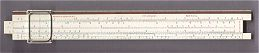Originally consisting of A, B, C, D scales, most modern versions of this type of rule also include an inverted C scale (CI) on the front of the slide and a cubic (K) scale at the base of the stator (also known as the body, base or stock of the rule). On the reverse of the slide, you will commonly find sine (S) and tan (T) trig scales and the log (L) scale. Common rules with this arrangement would include the K&E 4053 (illustrated on the right) and Post 1447. Many versions (like the 4053) also come with inch or centimeter rulers, adding to their usefulness.

Rietz

Front: K A [ B CI C ] D L
Back [S ST T ]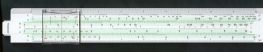Similar to the Mannheim arrangement, but with a few differences in the location of scales. In fact, it's probably a more logically consistent design, as the cubic (K) scale is located above the squared A/B scales, and the log (L) scale is now on the face of the rule instead of with the trig scales. This design also allowed the inclusion of the extended sine scale (ST), useful for both sines and tans. Common on many German-made slide rule models, such as my Faber 57/87 (illustrated) and Eco Bra 1461. These rules also frequently possessed standard rulers as well.

Darmstadt

Front: K A [ B CI C ] D P
Back: [ LL1 LL2 LL3 ]
Hard Edge: S T
Slanted Edge: L
Alternate "Advanced" arrangement: S and T scales added to front stator, and L and LL0 scales added to back of slide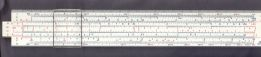Another German innovation, and one of my favourite designs due to its power and versatility. The original Darmstadt design was mainly just the addition of log-log scales on the back of the slide and the interesting Pythagorean (P) scale on the face (see my Faber 1/54 or Nestler 0210 for examples). This required moving the sine S and tan T scales to the hard edge and the log L scale to the slanted edge, which is an unsual place for them (requiring a translating cursor mark). Later on, an "advanced" Darmstadt design was developed with the alternate scale arrangement shown above (illustrated by my Hemmi 130W, shown on the right). Basically, they moved the S and T scales to the face of the rule (along with a rather useless inverted BI scale) and put the standard log L scale on the back with the other log-log scales. This was a rather unusual change, and is actually more reminiscent of the German practice of putting trig scales on the stator face of duplex rules (Japanese duplex rules typically followed the American custom of leaving them on the back of the slide). See below for a comparison of duplex rules. In any case, this type of desktop model also had the advantage of frequently coming with standard rulers.

## Duplex models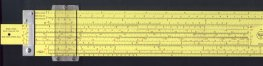All duplex models feature scales on both sides of the stators and slide, with a dual-faced cursor that can reliably relate each side to the other. This greatly increases the number of scales that can be used in combination, although it also potentially increases the risk of error due to misalignment or unintended movement during manipulation. Arrangement of scales differed considerably from model to model, but certain general characteristics were commonly present. Most rules featured additional log-log scales for exponential power calculations (e.g. LL01, LL3, etc.), needed for solving non-integer exponent problems. They also featured C/D scales "folded" at the value of pi (i.e. multiplied by 3.14159...) that are very useful for calculations involving circles and spheres (e.g. CF, DF, CIF, etc.). Some also included expanded square root scales, designated as R1/R2 or Sq1/Sq2, or additional hyperbolic trig scales (e.g. Sh1/Sh2, Th) on "vector" models. Although the variations are too numerous to mention individually, most people tend to have their own favourite arrangement and maker. For comparison, check out some my favourite high-end rules, like the Hemmi-made Versalog, the Nestler-made Staedtler 544 28, the Pickett N4-ES (illustrated), and the K&E 4081.

One interesting note on duplex designs, I've noticed that most American (e.g. K&E, Dietzgen) and Japanese makers (e.g. Hemmi, Relay) liked putting the log-log exponent scales on the front stators, with the trig scales on the back of the slide. This trig placement is similar to the design of most desktop models, which commonly put those scales on the back of the slide. However, German makers (e.g. Faber, Aristo, Nestler) invariably liked putting the log-log scales on the back of the stators, and the trig scales on the front stators. In contrast, many English models featured trig scales on the front of the slide, as do high-end Pickett rules (along with their base 10 log-log scales ... I get the feeling they just liked being different!). Obviously, there's a certain amount of live-and-let-live involved here, but there may be certain advantages to one arrangement or the other, depending on how you plan to use the rule. Personally, I'm not clear about the rationale behind these preferences, so if anyone has any thoughts, please drop me a line. For those of you who are interested, Ron Manley has a section on his The A to Z of Slide Rules page explaining how to use the trig scales in each of these arrangements. Incidentally, like most people, I tend to identify the "front" of a duplex rule by where the maker and model identification labels or logos are placed, which also generally corresponds to the location of the primary C/D scales.

## Circular models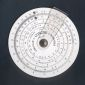Circular slide rules offer the advantage increasing scale length in a compact space by arranging the scales in a circle instead of on a straight line. At their simplest, they consist of a round disk with dual movable cursors that act sort of like dividers on a Gunter's scale. More commonly, they have a second rotating disk and a single cursor, much the like the common stator/slide/cursor action of a linear slide rule. A good example of a common circular rule is my Concise 28N (illustrated on the right). Circular rules can also be duplex in design, with an additional rotating disk and translating cursor on the back. They were never as popular as linear rules, probably due to the difficulty of keeping the disks and cursors steady while doing manipulations or taking readings. Many circular slide rules are available as additions to some basic flat device or printed table, as evidenced by the E6-B flight computer used by pilots. Various types of cylindrical slide rules have also used throughout history, including the pocket model Otis King.

# Common Slide Rule Scales

This list is hardly exhaustive, and readers are referred to the other relevant slide rule resources listed on my Links and Books pages. Any omissions or errors presented here are entirely of my own doing, and I welcome any comments or additional suggestions readers may have. For detailed instructions on how to use a slide rule, please see my Slide Rule Manuals page.

 Scale Label ValueRelative to C/D Description of Use A X2 Scale of Squares. Typically found on the top stator, and used in conjunction with the D scale on the bottom stator. The squared value of the D scale can be read off the A scale. Similarly, square roots of the A scale can be read off the D scale. In this latter case, however, the number of digits has to be considered in order to use the right portion of the scale (see the R1/R2 scales further down this page for comparison). Scale units typically range from 1 to 100. B X2 Scale of Squares. Found on the slide, and used in conjunction with the C scale to read the square/square root values just as for the A/D scales. C X Fundamental Slide Rule scale. Located on the slide, most calculations are done in conjunction with the fundamental C/D scales. Runs from 1 to 10 (or 0.1 to 1, or 10 to 100, etc. depending on your point of view) in the basic log scale format that is fundamental to a slide rule. CF X x pi Folded C scale. Usually begins at the value of pi (i.e. 3.14159...), and is used for quickly doing multiplication or division problems involving pi (i.e. scale is considered "folded" at value of pi). Located on the slide, and is useful for calculations involving circles and spheres without having to reset the index (recall: pi is the ratio of the circumference of a circle to its diameter). Typically only found on duplex rules. CF/M X x loge10 Folded C scale. Begins at the value of 2.30, which is the log to base e of 10. Used on high-end Pickett duplex rules like the N4, which has log-log scales in base 10 format instead of the common base e natural log. This folded scale allows you to quickly convert results from base 10 system to base e (i.e. if you have the value of log to base 10 on the C/D scales, the equivalent log to base e value would directly correspond to the CF/M or DF/M scale). Not to be confused with the DFM scale found on other Pickett rules that use the common base e log-log scales (see further down this page). CI 1 / X Reciprocal C scale. Gives the reciprocal values of C (i.e. read "backwards", or 10 to 1, from standard C scale). Located on slide, the CI scale is useful for simplifying division calculations, or in cases where the slide would extend too far from the body of the rule using the C/D scales. CIF 1 / (pi x X) Reciprocal Folded C scale. Located on the slide, gives the reciprocal values of the CF scale. Typically only found on high end duplex rules, and useful as an aid in doing calculations involving pi. D X Fundamental Slide Rule Scale. Located on the stator, most calculations are done in conjunction with the fundamental C/D scales. Like the C scale, units typically range from 1 to 10. DF X x pi Folded D scale. Identical to CF scale, beginning at the value of pi and located on the stator. Used on duplex rules for calculations involving pi. DF/M X x loge10 Folded D scale. Identical to CF/M scale, beginning at the value of 2.30, which is the log base e of 10. Used in conjunction with C/D to quickly convert base 10 log values to base e log values (commonly found on Pickett rules that use base 10 log-log scales). See earlier entry for CF/M for more detail, and contrasting for DFM for comparison. DFM X x log10e Folded D scale. Similar to DF/M scale, only now folded at 0.4343 which is the log to base 10 of e (i.e. the reciprocal of log to base e of 10 which is used in the DF/M scale). Used on Pickett rules with standard base e log-log scale, and simply facilitates conversions between base e and base 10 log values. Frequently confused with CF/M and DF/M scales, but differentiated by division slash in label (i.e. "M" is the log to base e of 10 and "/M" is the reciprocal, or 1/M, which is equivalent to the log to base 10 of e). A potentially useful scale in its own right, but issue confused due to DF/M scale needed on base 10 log-log scale rules DI 1 / X Reciprocal D scale. Identical to CI scale, located on stator. Gives the reciprocal values of D. Fairly uncommon, although seen on some high end duplex rules. DIF 1 / (pi x X) Reciprocal Folded D scale. Located on the stator, gives the reciprocal values of the DF scale. Considerably rare. E ex Log-log scale. Another designation for the exponential power scale commonly referred to as LL3 (see further down this page for all the LL scales). H - Horizontal Angles scale. Found on Stadia surveying slide rules for horizontal angle calculations. Used in conjunction with HC (horizontal distance), V (vertical angles), and R scales on Stadia rules. H1, H2 Square root of (1+X2) Hyperbolic scales. Although clearly related to trigonometric calculations, I'm unclear on how these scales were actually used in practice (see Sh and Th scales for comparison of hyperbolic vector scales). H1 typically ranges from 1.005 to 1.5, an H2 ranges from 1.5 to 10, although with some variation where one scale ends and the other begins. HC - Horizontal Distance scale. Found on Stadia surveying slide rules for horizontal distance calculations. Used in conjunction with H (horizontal angles), V (vertical angles), and R scales on Stadia rules. K X3 Scale of Cubes. Located on the body, used in conjunction with D scale to determine cubes and cube roots similar to A/B scales. Commonly found on many types of rules, with a scale range from 1 to 1000. Similar difficulty exists in determining cube root as with square roots on A/B scales, namely the actual result depends on number of digits in cubed value. KZ X x 360 Folded Fundamental scale. Used in conjunction with related fundamental Z scale on German Merchant slide rules, this scale is "folded" at 360. Apparently used on business (or "Kaufmann") slide rules for facilitating interest calculations based on the old banking standard of 360 days in a year (KZ refers to "Kapital Zins", or interest on the principal). See also the related Z, T, and p% scales (and thanks to Cyril Catt for the translations). Seems to me more useful today for facilitating calculations involving degree angles or conversions involving second/hour and related changes. L log X Log scale. Linear scale used to determine the log values (mantissa only) of other scales. Commonly used on many types of rules in conjunction with the C/D scale to determine the mantissa of a given number (log to base 10). Lg log X Log scale. Another designation for common base 10 log (L) scale. Ln ln X Natural Log scale. Used to determine the natural log values (ln, or log to base e) of numbers from other scales. Fairly uncommon, as most slide rule scales use base 10 logs (except for the log-log scales). LL0 e0.001x Log-log scale. Exponential power scale used for raising to a power that is greater than one, with a range of 1.001 to 1.01 in this case. The various log-log scales listed below are useful for power calculations more complicated than basic square (A/B) and cube (K) scales (i.e. they allow the raising non-integer numbers to non-integer powers). However, they are more complicated to use as the appropriate scale must be read, depending on the situation. Note, incidentally, that on high-end Pickett rules the log-log scales often refer to base 10 instead of base e, and thus go up to LL4 with different ranges per scale. LL1 or ZZ1 e0.01x Log-log scale. Exponential power scale used for raising to a power that is greater than one. Range of 1.01 to 1.1. See other log-log scales on this page for comparison. LL2 or ZZ2 e0.1x Log-log scale. Exponential power scale used for raising to a power that is greater than one. Range of 1.1 to e (i.e. 2.71828...), although there is some variation in the upper and lower bounds. LL3 or ZZ3 ex Log-log scale. Exponential power scale used for raising to a power that is greater than one. Range of e to 105, but with considerable variation in the upper limit. Sometimes referred to as simply "E". LL00 or LL/0 e-x Log-log scale. Exponential power scale used for raising to a power that is less than one. Range of 0.999 to 0.99. Frequently present with the additional scales listed below. LL01 or LL/1 e-0.1x Log-log scale. Exponential power scale used for raising to a power that is less than one. Range of 0.99 to 0.91 LL02 or LL/2 e-0.01x Log-log scale. Exponential power scale used for raising to a power that is less than one. Range of 0.91 to 1/e (i.e. roughly 0.37). LL03 or LL/3 e-0.001x Log-log scale. Exponential power scale used for raising to a power that is less than one. Range of 1/e to 0.0001, although variable at upper bound. M log X Log scale. Another designation for common base 10 log (L) scale. Symbol likely refers to "mantissa". P Square root of (1-X2) Pythagorean scale. Found on the stator, used to more accurately determine the cosine from the sine (S) scale for small angles. Can also be used more generally to calculate the square root of ( 1 - X2 ) when used in conjunction with D and A scales. Typically found on Darmstadt style rules. Scale ranges typically from 0.995 to 0. p% - Percentage scale. Found on the slide of Merchant or "kauffmann" type slide rules. Used in conjunction with the Z, KZ, and T scales to calculate interest rates (see other Merchant scales on this page). P1, P2 Square root of (1-X2) Pythagorean scale. P1 is identical to the P scale listed above. P2 is an extended P scale ranging from 0.99995 to 0.995. R 1 / X Reciprocal C scale. Typically another designation for the CI scale (see further up this page). Can also refer to a square scale (A) on Stadia surveying slide rules, usually in the range of 10 to 1000, and used in conjunction with Stadia H, HC, and V scales. R1, R2 Square root of X Scale of Square Roots. Read in conjunction with the D scale, used to quickly and easily determine square roots. If the number has an odd number of digits, then the odd-numbered R1 scale is used. If the number has an even number of digits, then R2 is used. Easier to remember than using A/B scales for square roots. R1 scale ranges from 1 to square root of 10 (i.e. 3.16228...), and R2 ranges from square root of 10 to 10 (basically a D scale "stretched out" over two scales). S sin X Scale of sines and cosines. Used to determine sines or cosines of angles, typically within range of 5.7 and 90 degrees (although there is some variation in lower limit). Often presented with increasing numbers for sines and decreasing numbers for cosines on the same scale. Found on many types of rules. Read in conjunction with the C/D scales, in values ranging from 0.1 to 1.0. Sh1, Sh2 sinh X Hyperbolic scale of sines. Typically used for electrical engineering functions and for defining the shape of a catenary curve. As such, generally found only on specialized high end duplex "vector" slide rules. Based on powers of exponents, often present with the tanh (Th) scale. Sh1 typically ranges from 0.1 to 0.89, and Sh2 ranges from 0.89 to 3.0, although there is considerable variation where each scale leaves off and the other begins. In absence of hyperbolic scales, calculations could still be made with exponential powers scales (i.e. log-log, or LL) with appropriate formulas. Sq1, Sq2 Square root of X Scale of Square Roots. Another designation for the R1/R2 scales for square roots (see above). SRT sin X, tan X Scale of sines and tangents. Another designation for the ST scale (see below). R refers to radians. ST sin, tan X Scale of sines and tangents. An extension of the S scale for small angles, typically used to determine sines and tangents of angles between 0.57 and 5.7 degrees (with some variation in upper and lower bounds). Since sines and tangents are nearly equal in this range, a single scale is used for both. Read in conjunction with C/D scales, in values ranging from 0.01 to 0.1. T tan X, cotan X Scale of tangents and cotangents. Used to determine tangents and cotangents of angles typically between 5.7 and 45 degrees on simple rules, and up to about 84.5 on rules that have the scale continuing "backwards" along the bottom length. When done this way, the base scale of up to 45 degrees typically relates tan X to the C or D scale (values representing 0.1. to 1), and the scale from 45 up to 84.5 relates tan X to the CI scale with the same range. Alternatively, cotan X can be determined using values 1 to 10 on the C/D and CI scales using the same method. Sometimes the scales may be broken up into two separate scales for greater clarity, as detailed below. On German Merchant slide rules, T can also refer the C/CF scales, and is used to calculate days (or "Tage") in interest calculations in relation to Z, KZ, and p% scales. T1, T3 tan X, cotan X Scale of tangents and cotangents. Identical to simple T scale (see above), running from angle degrees of approximately 5.7 to 45. Different manufacturers used different identifiers, but all T, T1, and T3 labels represent the same scale length and function (i.e. tan X to C or D scale, ranging from 0.1 to 1, and cotan X to C or D using values of 1 to 10). When labelled in this form, often presented in conjunction with a separate T2 scale for the remaining degrees of 45 up to 84.5. T2 tan X, cotan X Scale of tangents and cotangents. Used in relation with T1 (also labelled T and T3) scale above for remaining degree angles of 45 up to 84.5 (typically). Advantage of this separation method is that it doesn't require a CI scale on the same face. Th tanh X Hyperbolic scale of tangents. Used along with Sh scales in electrical engineering calculations (common on vector slide rules). Typically covers the range of 1 to 3. V Volts Voltage scale. Typically found on electrical rules for calculating volt drop in conductors. Also seen on Stadia surveying slide rules for vertical angles, used in conjunction with Stadia H, HC, and R scales. W1, W2 Square root of X Scale of Square Roots. Another designation for the R1/R2 scales for square roots (see earlier on this page). Z X Fundamental Slide Rule scale. Another designation for the standard C/D scales used on German Merchant slide rules, in conjunction with the folded KZ, T, and p% scales (see earlier on this page). Apparently, Z refers to "Zins", or interest. ZZ1, ZZ2, ZZ3 ex Log-log scale. Another designation for the exponential power scale (log-log scale) used for raising to powers greater than one. See LL scales listed earlier on this page for more info.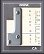Return to Eric's Slide Rule Page

Page last updated on September 12, 2002 -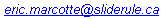All material © 1999, 2002 by Eric Marcotte, Ph.D.